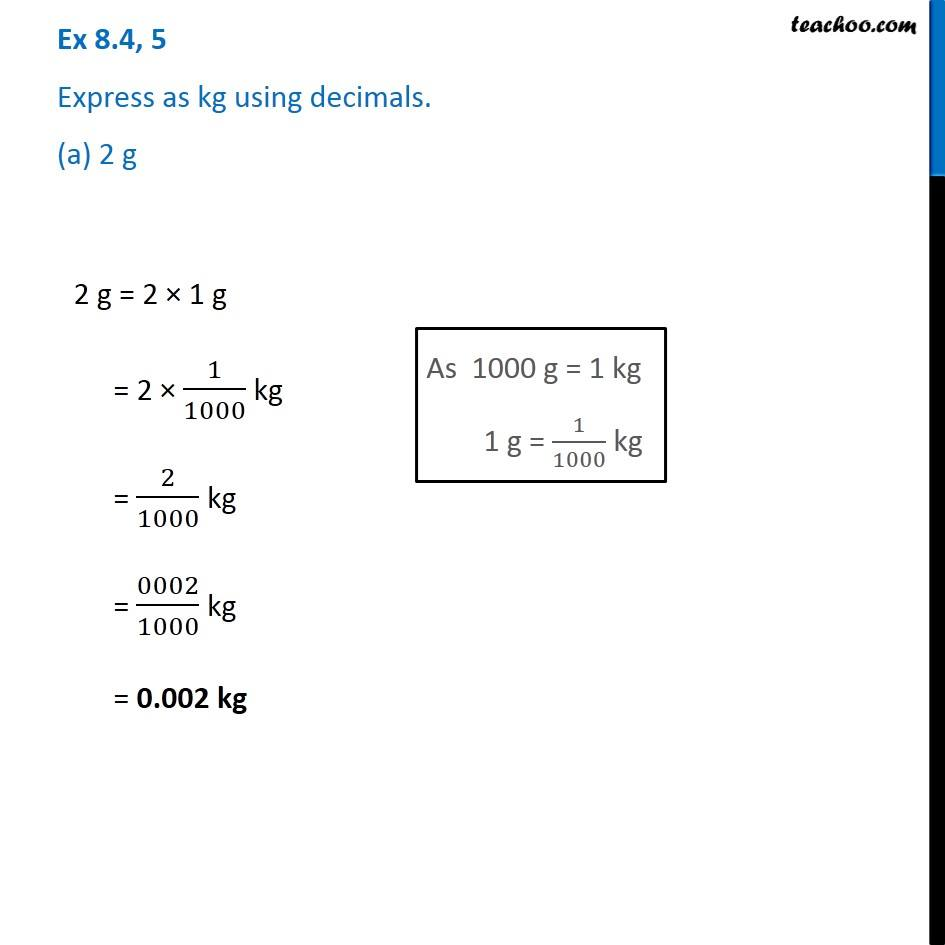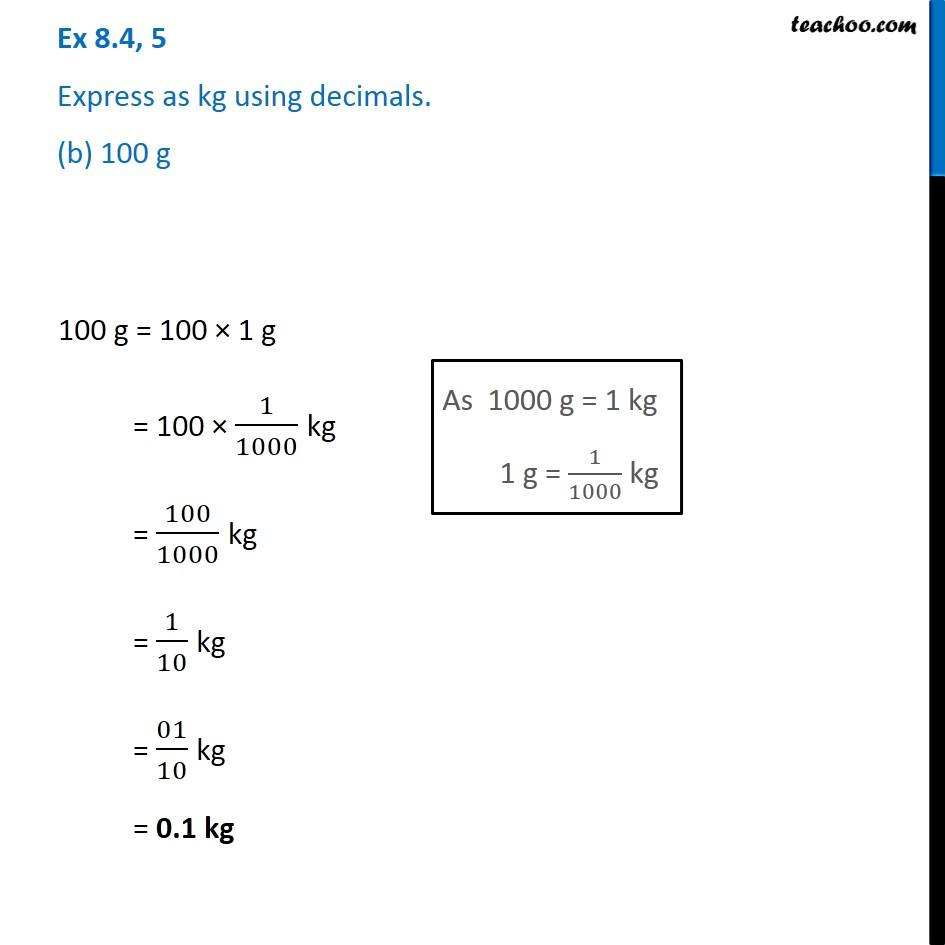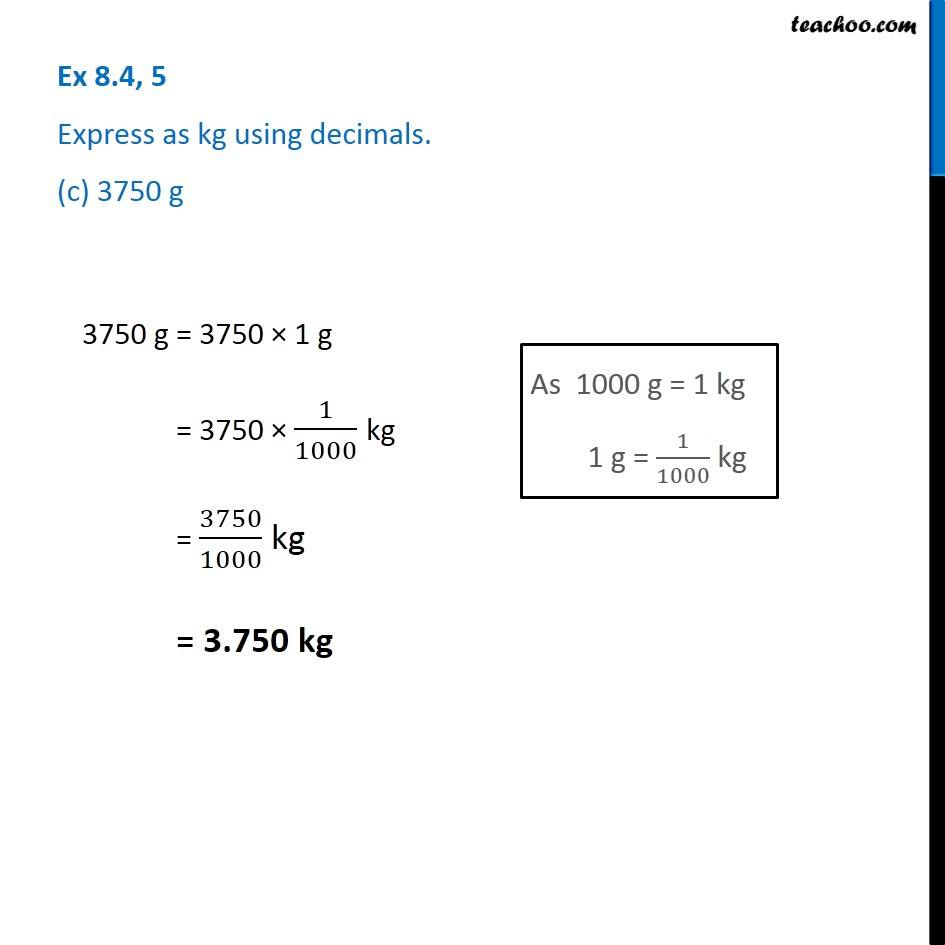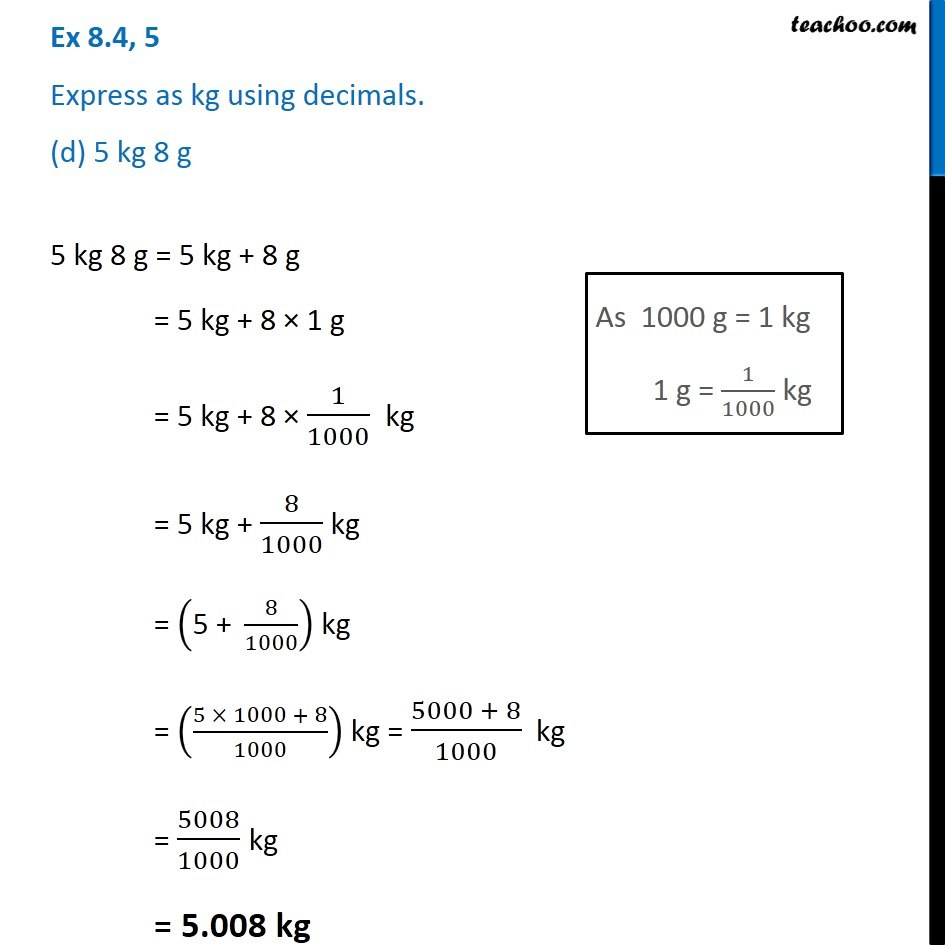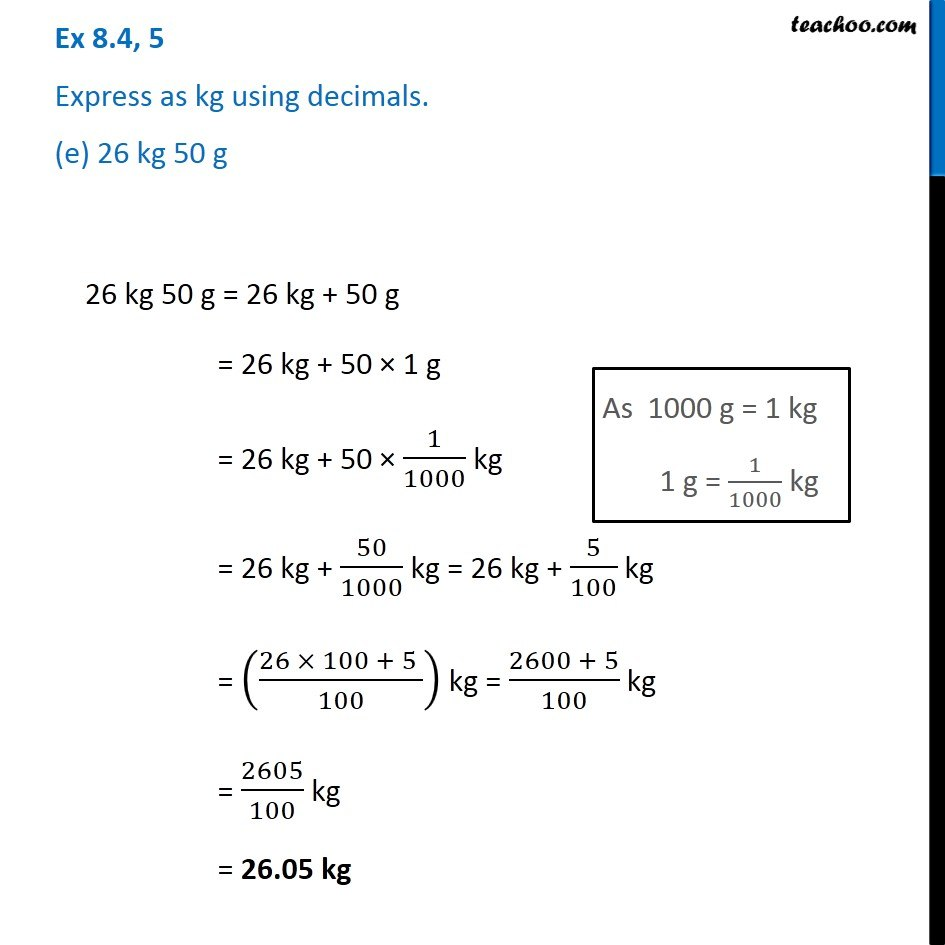1. Chapter 8 Class 6 Decimals
2. Serial order wise
3. Ex 8.4

Transcript

Ex 8.4, 5 Express as kg using decimals. (a) 2 g 2 g = 2 × 1 g = 2 × 1/1000 kg = 2/1000 kg = 0002/1000 kg = 0.002 kg Ex 8.4, 5 Express as kg using decimals. (b) 100 g 100 g = 100 × 1 g = 100 × 1/1000 kg = 100/1000 kg = 1/10 kg = 01/10 kg = 0.1 kg Ex 8.4, 5 Express as kg using decimals. (c) 3750 g 3750 g = 3750 × 1 g = 3750 × 1/1000 kg = 3750/1000 kg = 3.750 kg Ex 8.4, 5 Express as kg using decimals. (d) 5 kg 8 g 5 kg 8 g = 5 kg + 8 g = 5 kg + 8 × 1 g = 5 kg + 8 × 1/1000 kg = 5 kg + 8/1000 kg = ("5 + " 8/1000) kg = ((5 × 1000 + 8)/1000) kg = (5000 + 8)/1000 kg = 5008/1000 kg = 5.008 kg Ex 8.4, 5 Express as kg using decimals. (e) 26 kg 50 g 26 kg 50 g = 26 kg + 50 g = 26 kg + 50 × 1 g = 26 kg + 50 × 1/1000 kg = 26 kg + 50/1000 kg = 26 kg + 5/100 kg = ((26 × 100 + 5 )/100) kg = (2600 + 5)/100 kg = 2605/100 kg = 26.05 kg

Ex 8.4

Chapter 8 Class 6 Decimals
Serial order wise